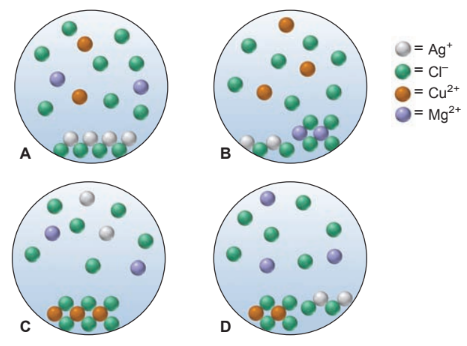### Solution Found!# Solved: A chemist mixes solid AgCl, CuCl2, and MgCl2 in enough water to give a final

Chapter 4, Problem 4.133

(choose chapter or problem)

Get Unlimited Answers! Check out our subscriptions
QUESTION:

A chemist mixes solid $$\mathrm{AgCl}$$, $$\mathrm{CuCl}_2$$, and $$\mathrm{MgCl}_2$$ in enough water to give a final volume of 50.0 mL.

(a) With ions shown as spheres and solvent molecules omitted for clarity, which of the following best represents the resulting mixture?(b) If each sphere represents $$5.0\times10^{-3}$$ mol of ions, what is the total concentration of dissolved (separated) ions?

(c) What is the total mass of solid?

QUESTION:

A chemist mixes solid $$\mathrm{AgCl}$$, $$\mathrm{CuCl}_2$$, and $$\mathrm{MgCl}_2$$ in enough water to give a final volume of 50.0 mL.

(a) With ions shown as spheres and solvent molecules omitted for clarity, which of the following best represents the resulting mixture?(b) If each sphere represents $$5.0\times10^{-3}$$ mol of ions, what is the total concentration of dissolved (separated) ions?

(c) What is the total mass of solid?

Step 1 of 4

Plan: Check the solubility rules if the compounds are soluble or insoluble. Any insoluble ionic compound(s) will appear as solid in the correct scene, while any soluble ionic compound(s) will appear as dissociated ions. Find molarity by dividing moles by the volume of the solution in liters.